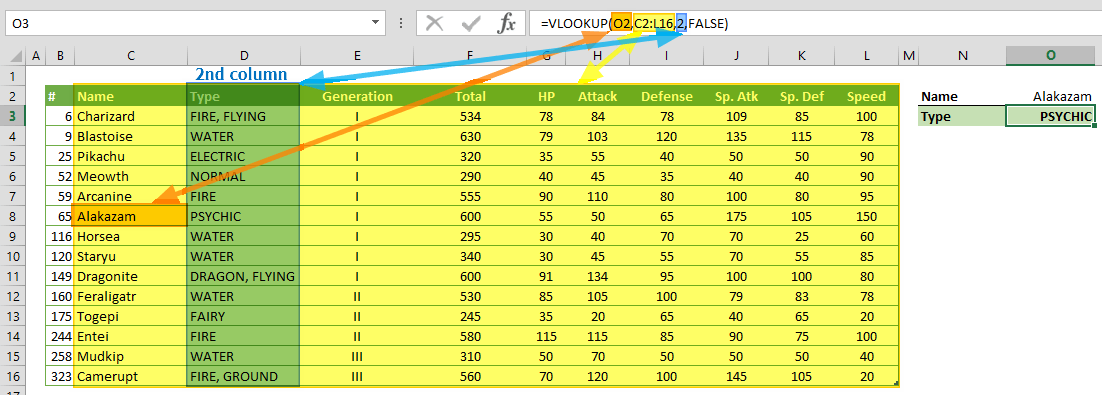VLOOKUP function is a lookup and reference function that can search a specific information in a table. In this guide, we’re going to show you how to use the VLOOKUP function and also add some tips and error handling methods.

The VLOOKUP searches a value in the first column of a range and returns the value in specified column if searching is successful.

# Supported versions

• All Excel versions

# VLOOKUP Syntax

VLOOKUP(lookup_value, table_array, col_index_num, [range_lookup])

# Arguments

 lookup_value What you are looking for table_array Where you are looking; range where you want to look up the value col_index_num What we want to know; the column number in the range containing the return value [range_lookup] Optional. Whether we want to be precise or approximate in our search; indicated as 0/FALSE or 1/TRUE. Default value is 1/TRUE.

Examples

## Example 1

=VLOOKUP(O2,C2:L16,2,FALSE)
formula searches value of cell O2 ("Alakazam") in first column of the table C2:L16. When [range_lookup] argument is FALSE, the function assumes that the searched value exists in the first column exactly. If the formula finds the value, it returns the value in 2nd column of matched row.

Note: The VLOOKUP function is not case-sensitive. So, "Alakazam" and "ALAKAZAM" criteria will work the same.## Example 2

=VLOOKUP(O5,B2:L16,3,TRUE)
formula searches value of cell O2 ("145") in first column of the table B2:L16. When [range_lookup] argument is TRUE, the function assumes that the first column is sorted either numerically or alphabetically. The formula returns the value in 3rd column of matched row, if it finds the closest value.# Tips

• Always use a sorted list when [range_lookup] argument is omitted, or set TRUE
• Wildcards can be used for searching text values. Searching a wild card in a range of numeric values may return incorrect results.
 Operator Description Criteria Sample Criteria Meaning ? Takes the place of a single character “Ala?azam” 8-character word starts with “Ala” and finishes with "azam" * Can take the place of any number of characters. “Ala*” Any number of character word starts with “Ala” ~ Use tilde in front of a  question mark or an asterisk to actually find them “Ala~*” Equal to "Ala*"

# Common Issues

## Wrong Value

If [range_lookup] is omitted or set TRUE, check whether your first column is sorted or not. If you need to search for an exact match with the values in the first column, set [range_lookup] to FALSE.

## #N/A!

### [range_lookup] = TRUE

If search value is smaller than the smallest value in the first column, you get #N/A! error.

### [range_lookup] = FALSE

If search value doesn't match any values in the first column, you will get #N/A! error.

## #VALUE!

If col_index_num is smaller than 1, you will get #VALUE! error.

## #REF!

If col_index_num is higher than the column number of table_array, you will get #REF! error.